# TARGET SBI EXAM 2018: QUANTITATIVE- PERCENTAGE 3

Dear Bankersdaily Aspirants,

Hope , Your preparation for SBI Clerk & PO exam 2018 is good . Since SBI Clerk & PO exam is a Two level which will be conducted in an online mode, Competition will be higher for this SBI Clerk & PO Exam and the most important thing , Current affairs section will be there in this exam. So aspirants have to concentrate in Four sections namely Aptitude, Reasoning, English, Current Affairs.

The person with high speed and very good accuracy only can crack this exam to taste the success. The candidates will be selected on the basis of performance in this online exam test. So the candidate with consistent hard work and regular practice will crack the exam very easily.

Here are some questions which will help you to identify your level of preparation.

Exam: SBI  Clerk & PO Exam 2018

Topic: PERCENTAGE 3

Timing: 7 minutes

1) In a certain school, 20% of students are below 8years of age. The number of students above 8 years of age is 2/3of the number of students of 8 years of age which is 48. What is the total number of students in the school?

a) 72

b) 80

c) 120

d) 150

e) 100

2) 3 years ago the population of a town was 1,60,000. In the three respective years the population increased by 4%, 5% and 8% respectively. What is the population of town after 3 years?

a) 1,88,697

b) 1,66,733

c) 1,76,736

d) 1,80,766

e) 1,69,766

3) Pankaj gave 50 percent of the amount to akash. Akash in turn gave two-fifth of the amount to venu. After paying a bill of 500 rupees, venu now have 8000 rupees left with him. Find the amount hold by pankaj initially.

a) 41500

b) 42500

c) 43500

d) 44500

e) none of these

4)  If A = x% of y and B = y% of x, then which of the following is true?

a) Relationship between A and B cannot be determined

b) A is smaller than B

c) None of these

d) If x is smaller than y, then A is greater than B

e) A is greater than B

5) The cost of raw material of a product increases by Rs 30, the manufacturing cost increases by Rs 20 and the selling price of the product increases by Rs 60. The raw material and the manufacturing cost, originally, formed Rs 40 and Rs 60 of the total cost respectively. If the original profit was one-fourth the original manufacturing cost, find the new profit percentage approximately.

a) 17.23%

b) 15.48%

c) 16.67%

d) 14.38%

e) 18.66%

6) If the price of petrol increases by 25% and Raj intends to spend only an additional 15% on petrol, by how much % will he reduce the quantity of petrol purchased?

a) 10%

b) 12%

c) 18%

d) 16%

e) 8%

7) B as a percentage of A is equal to A as a percentage of (A+B) . Find B as a percentage of A.

a) 73%

b) 82%

c) 62%

d) 96%

e) 99%

8) In a competitive examination in State ‘A’, 6% candidates got selected from the total appeared candidates. State ‘B’ had an equal number of candidates appeared and 7% candidates got selected with 80 more candidates got selected than State ‘A’. What was the number of candidates appeared from each state?

a) 8000

b) 8400

c) 7600

e) None of these

9) A part of sum of Rs. 20,000 is lent at 10% and the remaining such at 12% per annum. If the average rate of interest is 10.2%, then the two parts are

a) 2000, 18000

b) 18000, 2000

c) 15000, 7000

d) 9000, 7000

e) None of these

10) A shop keeper employed a servant at a monthly salary of Rs. 4000. In addition to it, he agreed to pay him a commission of 20% on the monthly sale. How much sale in rupees should the servant do if he wants monthly income as Rs. 8000?

a) 10,000

b) 20,000

c) 30,000

d) 40,000

e) None of these

1.e)

Let the number of students be x

No.of Students above 8 years of age = (100-20)% of x=80%of x

80% of x= 48+2/3 of 48

8x/10=48+32

8x/10=80

x=100

2.a)

Initial Population = 1,60,000

In first year, 4% increased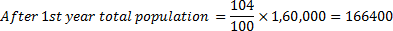In 2nd year , 5% increased

After 2 years the total population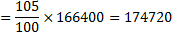In 3rd year, 8% increased

After 3 years the total population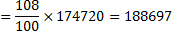3.b)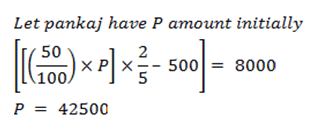4.c)

A=XY/100

B=XY/100

So A=B is a answer

So A=B is a answer

5.c)

manufacturing cost = Rs 60

Raw materials cost = Rs 40

Original Profit 1/4 of Manufacturing Cost = 15 rs

So original selling is price 60 + 40 + 15=  Rs 115

New raw material cost = 40 + 30 = 70

New manufacturing cost = 60 + 20 = 80

New cost of the product = 150

New selling price = 115 + 60 = 175

New profit percentage = (27/175)*100=16.666=16.7

6.e)

Let the price of 1 litre of petrol be Rs.x and let Raj initially buys ‘y’ litres of petrol.

Therefore, he would have spent Rs. xy on petrol.

When the price of petrol increases by 25%, the new price per litre of petrol is 1.25x.

Raj intends to increase the amount he spends on petrol by 15%.

i.e., he is willing to spend xy + 15% of xy = 1.15xy

Let the new quantity of petrol that he can get be ‘q’.

Then, 1.25x *q = 1.15xy

As the new quantity that he can buy is 0.92y, he gets 0.08y lesser than what he used to get earlier.

Or a reduction of 8%.

7.c)

B/A= ( A/(A/B))

B/A=X

X=  1/(1+(B/A))

X=1/(1+X)

solving we get x=0.62

x=62%

8.a)

Let the number of candidates appearing from each state be X.

Then,

7% of X – 6% of X = 80

Or, 1% of X = 80

Or, X = 8*100

X = 8000.

9.b)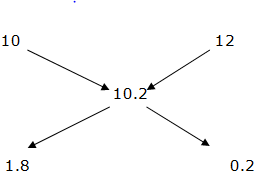First Part =(1.8/2)*20000

=0.9*20000=18000

Second Part = 20000-18000

Second Part = 2000

Second Part = 20000-18000

Second Part =2000

10.b)

8000-4000=4000

20% of 4000 =(4000/20) * 20

= 20,000

For the other Sections in the Day 3 of the TARGET SBI PLANNER , please check the below given links.

Aspirants can also check the other topics from the SBI CLERK STUDY PLANNER from the link given below. DO bookmark the given link as all the posts will be updated in that page and aspirants can check the daily updates from this page or from the Homepage of Bankersdaily.